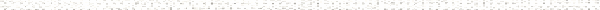A Precalculus Outline

 PrerequisitesObjective: FactoringObjective: Simplifying Rational ExpressionsObjective: Finding the Least Common Denominator - Rational ExpressionsObjective: Adding and Subtracting Rational ExpressionsObjective: Simplifying Compound (Complex) FractionsObjective: Definition of RadicalsObjective: Using the Product Rule for RadicalsObjective: Using the Quotient Rule for RadicalsObjective: Simplifying Radical ExpressionsObjective: Adding and Subtracting Radical ExpressionsObjective: Solving Equations Involving RadicalsObjective: Identifying Extraneous Solutions to Equations Involving RadicalsObjective: Definition of Rational ExponentsObjective: Simplifying Expressions with Rational ExponentsObjective: Soving Equations Involving Rational Exponents